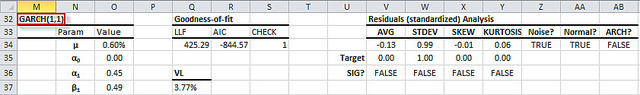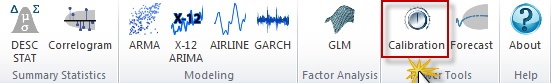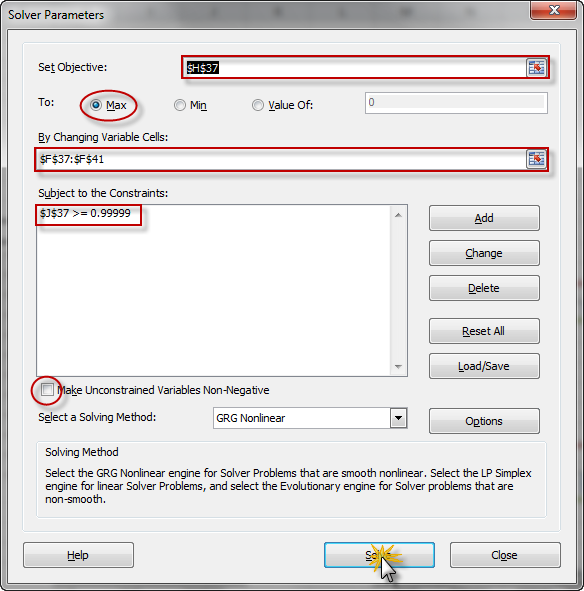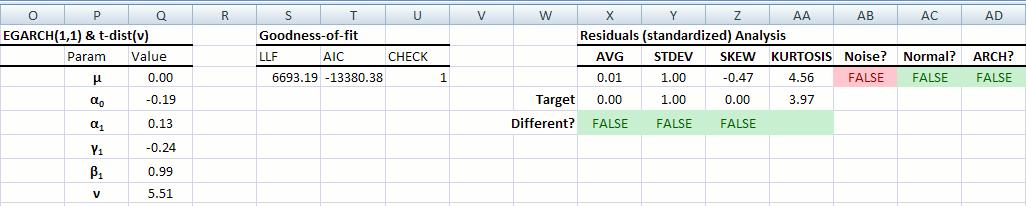# Model Calibration

The process of finding the Maximum Likelihood Estimation (MLE) of a model's parameters is referred to as model calibration. This process requires an optimizer to maximize the Log-Likelihood Function (LLF) by varying the model's parameters. Excel comes with a built-in Add-in called Solver which can be used for this purpose.

## Process

The Model calibration functionality invokes the Solver dialog box and initializes its parameters with the given model in the worksheet. To use this function, select the cell in your model that defines the model and its order (e.g. "GARCH(1,1)").Locate the calibration icon, and click on it.Notes:

1. The Solver Add-in should be installed on your system. To check, go to Excel's Add-ins manager and look for "Solver".
2. If the Solver is installed but deactivated, the calibration function will enable it.
3. The first cell in the mode is the one that describes the model and its order, e.g. the cell should read "ARMA(p,q)" or "GARCH(p,q)".
4. The calibration function assumes a model layout similar to the layout generated by ARMA/GARCH modeling. In particular:
• The LLF function should be in the 2nd row down and the 4th column to the right of the selected cell
• The CHECK function should be in the 2nd row down and the 6th column to the right of the selected cell
• The model's parameters are listed in your worksheet starting in the 2nd row down, the 2nd column to the right, and end before the first empty row

## Output